Students can Download Tamil Nadu 12th Chemistry Model Question Paper 5 English Medium Pdf, Tamil Nadu 12th Chemistry Model Question Papers helps you to revise the complete Tamilnadu State Board New Syllabus, helps students complete homework assignments and to score high marks in board exams.

## TN State Board 12th Chemistry Model Question Paper 5 English Medium

Time: 3 Hours
Maximum Marks: 70

Instructions:

1. The question paper comprises of four parts
2. You are to attempt all the parts An internal choice of questions is provided wherever applicable
3. All questions of Part I, II, III, and IV are to be attempted separately
4. Question numbers 1 to 15 in Part I are Multiple Choice Questions of one mark each. These are to be answered by choosing the most suitable answer from the given four alternatives and writing the option code and the corresponding answer
5. Question numbers 16 to 24 in Part II are two-mark questions. These are lo be answered in about one or two sentences
6. Question numbers 25 to 33 in Part III are three-marks questions. These are to be answered in about three to five short sentences
7. Question numbers 34 to 38 in Part IV are five-mark Questions These are to answered in detail. Draw diagrams wherever necessary

Part – 1

Answer all the questions. Choose the correct answer. [15 × 1 = 15]

Question 1.
In the extraction of aluminium from alumina by electrolysis, cryolite is added to …………..
(a) Lower the melting point of alumina
(b) Remove impurities from alumina
(c) Decrease the electrical conductivity
(d) Increase the rate of reduction
(a) Lower the melting point of alumina

Question 2.
Compound used for propellant is
(a) BN
(b) H2B4O7
(c) B2H6
(d) Borax
(c) B2H6Question 3.
P4O6 reacts with cold water to give …………..
(a) H3PO3
(b) H4P2O7
(c) HPO3
(d) H3PO4
(a) H3PO3

Question 4.
Which one of the following elements show high positive electrode potential?
(a) Ti2+
(b) Mn2+
(c) CO2+
(d) Cr2+
(c) CO2+

Question 5.
As per IUPAC guidelines, the name of the complex [Co(en)2(ONO)Cl]Cl is …………..
(a) chlorobisethylenediaminenitritocobalt(III) chloride
(b) chloridobis(ethane-l,2-diamine)nitro k-Ocobaltate(III) chloride
(c) chloridobis(ethane-l,2-diammine)nitrito k-Ocobalt(II) chloride
(d) chloridobis(ethane-l,2-diamine)nitro k-Ocobalt(III) chloride
(d) chloridobis(ethane-l,2-diamine)nitro k-Ocobalt(III) chloride

Question 6.
Solid NH3 solid CO2 are examples of …………………..
(a) Covalent solids
(b) polar molecular solids
(c) molecular solids
(d) ionic solids
(b) polar molecular solids

Question 7.
After 2 hours, a radioactive substance becomes$$\left(\frac{1}{16}\right)^{4}$$ of original amount. Then the half life ( in min ) is ………
(a) 60 minutes
(b) 120 minutes
(c) 30 minutes
(d) 15 minutes
(c) 30 minutes
Solution:Question 8.
What is the decreasing order of strength of bases?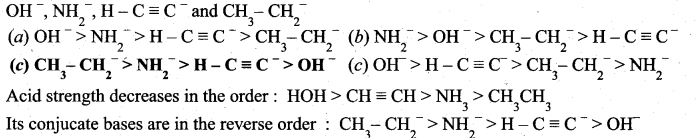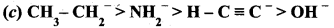Question 9.
Which electrolyte is used in Leclanche cell?
(a) ZnSO4 + CuSO4
(6) NH4Cl + ZnCl2
(e) NaCl + CuSO4
(d) MnSO4 + MnO2
(6) NH4Cl + ZnCl2

Question 10.
The phenomenon observed when a beam of light is passed through a colloidal solution is ………………
(a) Cataphoresis
(b) Eleætrophoresis
(c) Coagulation
(d) Tyndall effect
(d) Tyndall effectQuestion 11.
In the following sequence of reactions,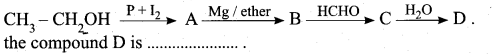(a) Butanal
(b) n-butyl alcohol
(c) propan-1-ol
(d) Propanal
(c) propan-1-ol

Question 12.
Of the following, which is the product formed when cyclohexanone undergoe’s aldol condensation followed by heating?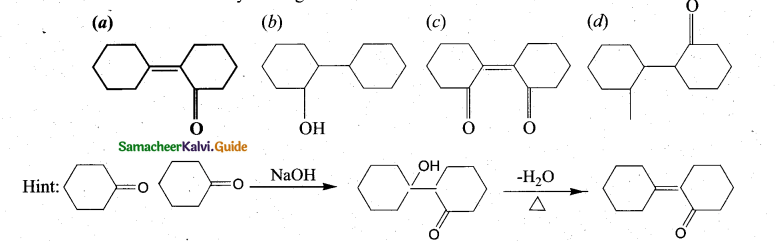(a)Question 13.(a) A – 2, B – 1, C – 4, D – 3Question 14.
Insulin, a hormone chemically is ………………..
(a) Fat
(b) Steroid
(c) Protein
(d) Carbohydrates
(c) Protein

Question 15.
The role of phosphate in detergent powder is
(a) control pH level of the detergent water mixture
(b) remove Ca2+ and Mg2+ ions from water that causes hardness of water
(c) provide whiteness to the fabric
(d) more soluble in soft water
(b) remove Ca2+ and Mg2+ ions from water that causes hardness of water

Part – II

Answer any six questions. Question No. 23 is compulsory. [6 x 2 = 12]

Question 16.
What are all the steps involved in metallurgical process?
The extraction of a metal from its ore consists the following metallurgical process.

• Concentration of the ore
• Extraction of crude metal
• Refining of crude metal

Question 17.
Give the uses of Borax.

• Borax is used for the identification of coloured metal ions.
• In the manufacture optical and borosilicate glass, enamels and glazes for pottery.
• It is also used as a flux in metallurgy and also acts as a good preservative.

Question 18.
Differentiate primary valency and secondary valency.
Primary Valency :

1. The primary valence of a metal ion positive in most of the cases and zero in certain cases.
2. The primary valence is always satisfied by negative ions.
3. The primary valences are non directional
4. Example: In COCl3.6NH3, the primary valence of cobalt is +3 Example: In CoCl3.6NH3, the secondary valence of cobalt +3

Secondary Valency :

1. The secondary valence as the coordination number.
2. The secondary valence is satisfied by negative ions, neutral molecular or positive ions.
3. The secondary valences are directional
4. Example: In COCl3.6NH3, the secondary valence of cobalt is 6Question 19.
Write a note about molecular solids.

• In molecular solids, the constituents are neutral molecules. They are held together by weak vander waals forces.
• Molecular solids are soft and they do not conduct electricity. Eg., Solid CO2

Question 20.
What are the limitations of Arrhenius concept?

• Arrhenius theory does not explain the behaviour of acids and base in non-aqueous solvents such as acetone, tetrahydro furan.
• This theory does not account for the basicity of the substances like ammonia which do not possess hydroxyl group.

Question 21.
Write a note on catalytic poison.
Catalytic poison: Certain substances when added to a catalysed reaction, decreases or completely destroys the activity of catalyst and they are often known as catalytic poisons.
For example, in the reaction, 2SO2 + O2 → 2SO3 with a Pt catalyst, the poison is AS2O3. i. e., AS2O3 destroys the activity of pt. AS2O3 blocks the activity of the catalyst. So, the activity is lost.

Question 22.
Convert phenyl magnesium bromide to phenyl methanol (or) How would you prepare phenyl methanol from Grignard reagent?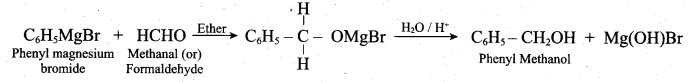Question 23.
Identify compounds A,B and C in the following sequence of reactions.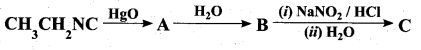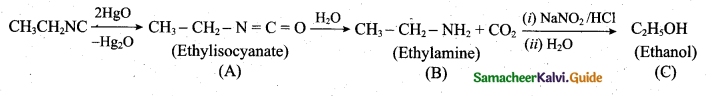Question 24.
Why cannot Vitamin C be stored in our body?
Vitamin C is water soluble, therefore it is readily excreted in urine and hence cannot be stored in the body.

Part – III

Answer any six questions. Question No. 29 is compulsory. [6 x 3 = 18]

Question 25.
All ores are minerals, but all minerals are not ores. Explain.
A naturally occurring substance obtained by mining which contains the metal in free state or in the form of compounds is called a mineral. In most of the minerals, the metal of interest is present only in small amounts and some of them contains a reasonable percentage of metal. Such minerals that – contains a high percentage of metal, from which it can be extracted conveniently and economically are called ores. Hence all ores are minerals but all minerals are not ores.

Question 26.
Discuss the Commercial method to prepare Nitric acid.
[OR]
How will you prepare nitric acid by Ostwald’s process?
Nitric acid prepared in large scales using Ostwald’s process. In this method ammonia from Haber’s process is mixed about 10 times of air. This mixture is preheated and passed into the catalyst chamber where they come in contact with platinum gauze. The temperature rises to about 1275 K and the metallic gauze brings about the rapid catalytic oxidation of ammonia resulting in the formation of NO, which then oxidised to nitrogen dioxide.

4NH3 + 5O2 → 4NO + 6H2O + 120 kJ
2NO + O2 → 2NO2

The nitrogen dioxide produced is passed through a series of adsorption towers. It reacts with water to give nitric acid. Nitric acid formed is bleached by blowing air.

6NO2 + 3H2O → 4HNO3 + 2NO + H2O

Question 27.
Actinoid contraction is greater from element to element than the lanthanoid contraction, why?

• Actinoid contraction is greater from element to element than lanthanoid cintraction. The 5f orbitals in Actinoids have a very poorer shielding effect than 4f orbitals in lanthanoids.
• Thus, the effective nuclear charge experienced by electron in valence shells in case of actinoids is much more than that experienced by lanthanoids.
• In actinoids, electrons are shielded by 5d, 4f, 4d and 3d whereas in lanthanoids, electrons are shielded by 4d, 4f only. ,
• Hence, the size contraction in actinoids is greater as compared to that in lanthanoids.

Question 28.
What are the examples of first order reaction?
(i) Decompostion of dinitrogen pentoxide
2N2O5(g) → 2NO2(g) + 1/2 O2(g)

(ii) Decomposition of thionylchloride
SO2Cl2(g) → SO2(g) + Cl2(g)

(iii) Decompostion of H2O2 in aqueous solution.
H2O2(aq) → H2O(l) + 1/2 O2(g)

(iv) Isomerisation of cyclopropane to propene

Question 29.
Complete the following reaction.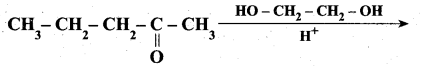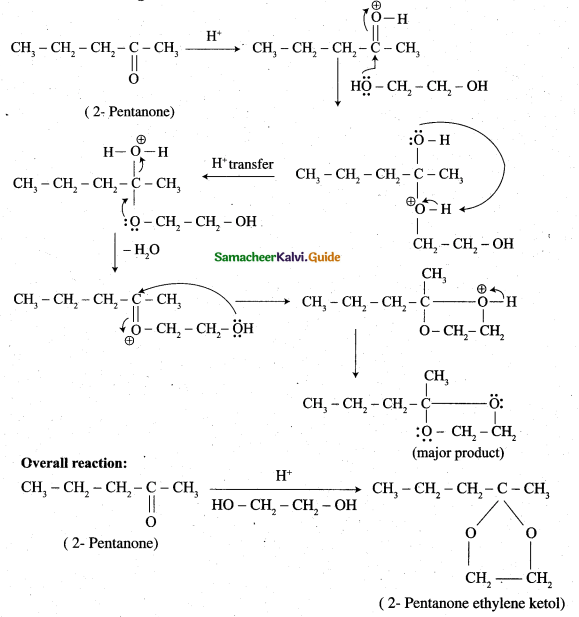Question 30.
How will you calculate degree of dissociation of weak electrolytes and dissociation constant using Kohlrausch’s law?
(i) The degree of dissociation of weak electrolyte can be calculated from the molar conductivity at a given concentration and the molar conductivity in infinite dilution using the formula $$\alpha=\frac{\Lambda_{\mathrm{m}}}{\Lambda_{\mathrm{m}}^{\circ}}$$

(ii) According to Ostwald’s dilution law $$\mathrm{K}_{\mathrm{a}}=\frac{\alpha^{2} \mathrm{C}}{1-\alpha}$$
Substituting α value in the above equation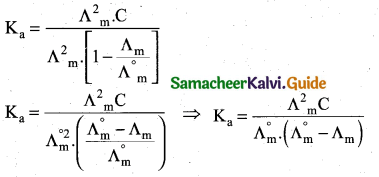Question 31.
What are the characteristics of adsorption?

• Adsorption can occur in all interfacial faces i.e., the adsorption can occur in between gas – solid, liquid – solid, liquid – liquid, solid – solid and gas – liquid. .
• Adsorption is always accompanied by decrease in free energy. When AG reaches zero, the equilibrium is attained.
• Adsorption is a spontaneous process.
• When molecules are getting adsorbed, there is always decrease in randomness of the molecules.
∆G = ∆H – T ∆S where ∆G = change in free energy
∆H = change in enthalpy
∆S = change in entropy
.’. ∆H = ∆G + T∆S
• Adsorption is exothermic and it is a quick process.
• If simultaneous adsorption and absorption take place, it is termed as ‘sorption’ and sorption of gases on metal surface is called occlusion.

Question 32.
What are the uses of cellulose?

• Cellulose is used extensively in manufacturing paper, cellulose fibres and rayon explosive.
• Gun cotton – nitrated ester of cellulose an explosive is prepared from cellulose.
• Cellulose act as food for animals.

Question 33.
How will you prepare PHBV? Give its use?
(i) The biodegrable polymer PHBV (Poly hydroxy butyrate-co hydroxyl valerate) is prepared by the polymerisation of monomers 3 – hydroxy butanoic acid and 3 – hydroxy pentanoic acid.(ii) It is used in orthopaedic devices and in controlled release of drugs.

Part – IV

Answer all the questions. [5 x 5 = 25]

Question 34.
(a) (i) Which type of ores can be concentrated by froth floatation method?
Give two examples for such ores. (2)
(ii) Explain the variation In E°M3+/M2+ series. (3)
[OR]
(b) (i) Mention the uses of silicon tetrachtoride. (2)
(ii) What are all the conditions that are necessary for catenation? (3)
(a) (i) Suiphide ores can be concentrated by froth floatation method.
e.g., (i) Copper pyrites (CuFeS2) (ii) Zinc blende (ZnS) (iii) Galena (PbS)

(ii)

• In transition series, as we move down from Ti to Zn, the standard reduction potential E° M3+/M2+value is approaching towards less negative value and copper has a positive reduction potential, i.e. elemental copper is more stable than Cu2+
• M3+/M2+ value for manganese and zinc are more negative than regular trend. It is due to extra stability arises due to the half filled d5 configuration in Mn2+ and completely filled d10 configuration in Zn2+.
• The standard electrode potential for the M3+ /M2+ half cell gives’the relative stability between M3+ and M2+.
• The high reduction potential of Mn3+ / Mn2+ indicates Mn2+ is more stable than Mn3+.
• For Fe3+/Fe2+ the reduction potential is 0.77 V, and this low value indicates that both Fe3+ and Fe2+ can exist under normal condition.
• Mn3+ has a 3d4 configuration while that of Mn2+ is 3d5. The extra stability associated with a half filled d sub-shell makes the reduction of Mn3+ very feasible [E° = +1.5 IV]

[OR]

(b) (i) Silicon tetrachloride is used in the production of semiconducting silicon.
It is used as a starting material in the synthesis of silica gel, silicic esters, a binder for ceramic materials.

(ii) Essential condition for catenation:

• The valency of elements is greater than or equal to two.
• Element should have an ability to bond with itself.
• The self bond must be as strong as its bond with other elements.
• Kinetic inertness of catenated compound towards other molecules.

Question 35.
(a) (i) Discuss the manufacture of chlorine. (3)
(ii) What is inert pair effect? (2)
[OR]
(b) (i) Calculate the magnetic moment of Ti3+ and V4+. (2)
(ii) Draw all possible geometrical isomers of the complex [CO(en)2Cl2]+ and identify the optically active isomer. (3)
(a) (i) Electrolytic process: When a solution of brine (NaCl) is electrolysed, Na+ and Cl ions are formed. Na+ ion reacts with OH ions of water and forms sodium hydroxide.

Hydrogen and chlorine are liberated as gases.Deacon’s process: In this process a mixture of air and hydrochloric acid is passed up a chamber containing a number of shelves, pumice stones soaked in cuprous chloride are placed. Hot gases at about 723 K are passed through a jacket that surrounds the chamber.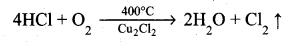The chlorine obtained by this method is dilute and is employed for the manufacture of bleaching powder. The catalysed reaction is given below,(ii) In p-block elements, as we go down the group, two electrons present in the valence s-orbital become inert and are not available for bonding (only p-orbital involves chemical bonding). This is called inert pair effect.

[OR]

(b) (i) Ti (Z = 22) Ti3+3d1
V (Z = 23) V4+ 3d1
.’. $$\mu=\sqrt{1(1+2)}=\sqrt{3}=1.73 \mu_{\mathrm{B}}$$ So they are paramagnetic.

(ii) 1. Cis – [Co(en)2Cl2]+The coordination complex [Co(en)2Cl2]+ has three isõmers two optically active cis forms and the optically inactive trans form.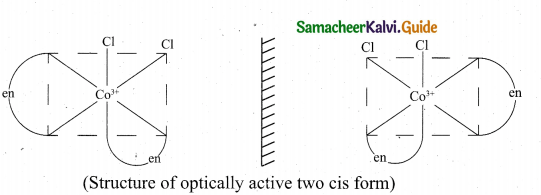Question 36.
(a) (i) Calculate the number of atoms in a fee unit cell.
(ii) How do nature of the reactant influence rate of reaction?
[OR]
(b) (i) Account for the acidic nature of HClO4
In terms of Bronsted – Lowry theory, identify its conjugate base.
(ii) IS it possible to store copper sulphate in an iron vessel for a long time?
Given $$\mathbf{E}_{\mathrm{Cu}^{2+} \mathrm{Cu}}^{\circ}=\mathbf{0 . 3 4 V}$$ and $$\mathbf{E}_{\mathbf{F e}^{2+} \mathbf{F e}}^{s}=+\mathbf{0 . 4 4 V}$$
(a) (i) Number of atoms in a fcc unit cell,(ii) Nature and state of the reactant:
We know that a chemical reaction involves breaking of certain existing bonds of the reactant and forming new bonds which lead to the product. The net energy involved in this process is dependent on the nature of the reactant and hence the rates are different for different reactants.
Let us compare the following two reactions that we carried out in volumetric analysis.

1. Redox reaction between ferrous ammonium sulphate (FAS) and KMnO4
2. Redox reaction between oxalic acid and KMnO4

The oxidation of oxalate ion by KMnO4 is relatively slow compared to the reaction between KMnO4 and Fe2+ . In fact heating is required for the reaction between KMnO4 and Oxalate ion and is carried out at around 60°C.

The physical state of the reactant also plays an important role to influence the rate of reactions. Gas phase reactions are faster as compared to the reactions involving solid or liquid reactants. For example, reaction of sodium metal with iodine vapours is faster than the reaction between solid sodium and solid iodine. Let us consider another example that we carried out in inorganic qualitative analysis of lead salts. If we mix the aqueous solution of colorless potassium iodide with the colorless solution of lead nitrate, precipitation of yellow lead iodide take place instantaneously, whereas if we mix the solid lead nitrate with solid potassium iodide, yellow coloration will appear slowly.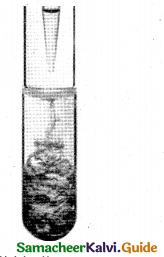[OR]

(b) (i) HClO4 ⇌ H+ + ClO4

According to Lowry – Bronsted concept, a strong acid has weak conjugate base and a weak acid has a strong conjugate base.

Let us consider the stabilities of the conjugate bases ClO4 , CIO3 , ClO2 and CIO formed from these acid HClO4, HClO3 , HClO2, HOCl respectively. These anions are stabilized to greater extent, it has lesser attraction for proton and therefore, will behave as weak base. Consequently the corresponding acid will be strongest because weak conjugate base has strong acid and strong conjugate base has weak acid.

The charge stabilization increases in the order, ClO < ClO2 < ClO3 < CIO4 . This means ClO4 will have maximum stability and therefore will have minimum attraction for H+. Thus ClO4 will be weakest base and its conjugate acid HClO4 is the strongest acid.

ClO4 is the conjugate base of the acid HClO4.

(ii) E0cell = E0ox + E0red = 0.44 V +0.34 V = 0.78 V
These +ve E0cell values shows that iron will oxidise and copper will get reduced i.e., the vessel will dissolve. Hence it is not possible to store copper sulphate in an iron vessel.

Question 37.
(a) (i) Explain the formation of water with copper catalyst by intermediate compound formation theory. (3)
(ii) O-nitro phenol is slightly soluble in water where as P-nitro phenol is more soluble. Give reason. (2)
[OR]
(b) (i) What happens when the following alkenes are subjected to reductive ozonolysis.
1. propene
2.1-Butene
3. Isobutylene (3)
(ii) What are reducing and non – reducing sugars? (2)
(a) (i) Formation of water due to the reaction of H2 and O2 in the presence of Cu can be given as(ii) O-nitro phenol is slightly soluble in water and more volatile due to intra molecular hydrogen bonding, whereas P-nitro phenol is more soluble in water and less volatile due to intermolecular hydrogen bonding.

[OR]

(b)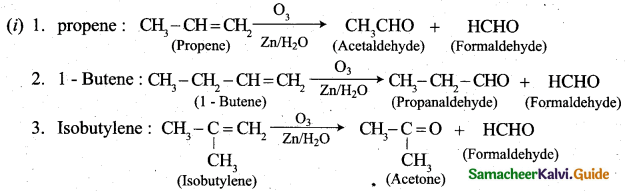(ii) Reducing sugars: Those carbonhydrates which contain free aldehyde or ketonic group and reduces Fehling’s solution and Tollen’s reagent are called reducing sugars. All monosacchaides whether aldose or ketone are reducing sugars.

Non-reducing sugars: Cabohydrates which do not reduce Tollen’s reagent and Fehling’s solution are called non-reducing sugars. Example Sucrose. They do not have free aldehyde group.

Question 38.
(a) A dibromo derivative (A) on treatment with KCN followed by acid hydrolysis and heating gives a monobasic acid (B) along with liberation of CO2 (B) on heating with liquid ammonia followed by treating with Br2 /KOH gives (C) which on treating with NaNO2 and HCl at low temperature followed by oxidation gives a monobasic acid (D) having molecular mass 74. Identify A to D. (5)
[OR]

(b) Explain the mechanism of cleansing action of soaps and detergents.(5)[OR]

(b) Mechanism of cleansing action of soaps and detergents:

• The cleansing action of both soaps and detergents from their ability to lower the surface tension of water, to emulsify oil or grease and to hold them in a suspension in water.
• This ability is due to the structure of soaps and detergents.
• In water a sodium soap dissolves to form soap anions and sodium cations. For example, the following chemical equation shows the ionisation of sodium palmitate.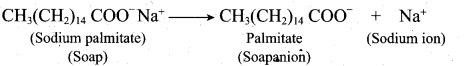• A soap anion consists of a long hydrocarbon chain with a carboxylate group on one end. The hydrocarbon chain, which is hydrophobic, is soluble in oils or grease. The ionic part is the carboxylate group which is hydrophilic, is soluble in water.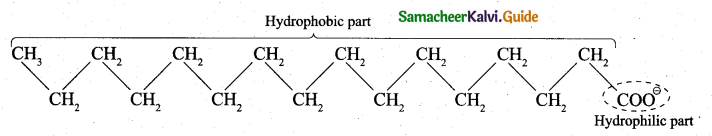• In water, detergent dissolves to form detergent anions and sodium cations. For example the following chemical equations show the ionisation of sodium alkyl sulphate and sodium alkyl benzene sulphate.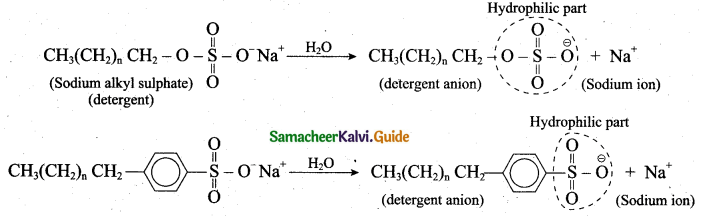6. The following explains the cleansing action of a soap or detergent on a piece of cloth with a greasy stain:

• A soap or detergent anion consists of a hydrophobic part and a hydrophilic part.
• Soap or detergent reduces the surface tension of water. Therefore the surface of the cloth is wetted thoroughly.
• The hydrophobic parts of the soap or detergents anions are soluble in grease.
• The hydrophilic parts of the anions are soluble in water.
• Scrubbing or mechanical agitation helps to pull the grease away from the cloth and the
• grease is broken into smaller droplets.
• Repulsion between the droplets causes the droplets to be suspended in water, forming an emulsion.
• Thus the droplets do not coagulate or redeposit on the cloth. Rinsing washes away the droplets.